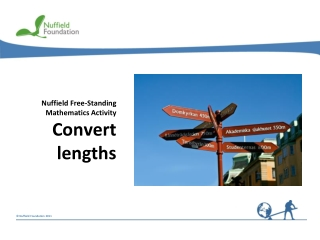DownloadDownload PresentationNuffield Free-Standing Mathematics Activity Convert lengths

# Nuffield Free-Standing Mathematics Activity Convert lengths

Download Presentation## Nuffield Free-Standing Mathematics Activity Convert lengths

- - - - - - - - - - - - - - - - - - - - - - - - - - - E N D - - - - - - - - - - - - - - - - - - - - - - - - - - -
##### Presentation Transcript

1. Nuffield Free-Standing Mathematics Activity Convert lengths

2. Convert lengths • How far are these towns in kilometres? • In metres? • In centimetres? • In millimetres? The purpose of this activity is to convert between the four metric units of length. Put the units of length in order of size biggest to smallest.

3. Let’s play bingo! Remember!1 km = 1000 m 1 m = 100 cm 1 cm = 10 mm 1 Choose a card. 2 As each length is called (or written on the board), look on your card for an equal length. If you find one, cover it up or cross it out. 3 The winner is the first person to cross out all the lengths on their card.

4. Dominoes 1 Shuffle, then share all of the dominoes between the players. 2 The first player puts down any domino. 3 Each player in turn tries to put down a domino next to a domino showing an equal length. 4 The first player to use all their dominoes wins.

5. At the end of the activity • How many metres are there in a kilometre? • How do you convert metres to centimetres? • How do you convert millimetres to metres? • How many millimetres are there in a centimetre? • Estimate how far you will walk today, in a week, in a year, in your lifetime.